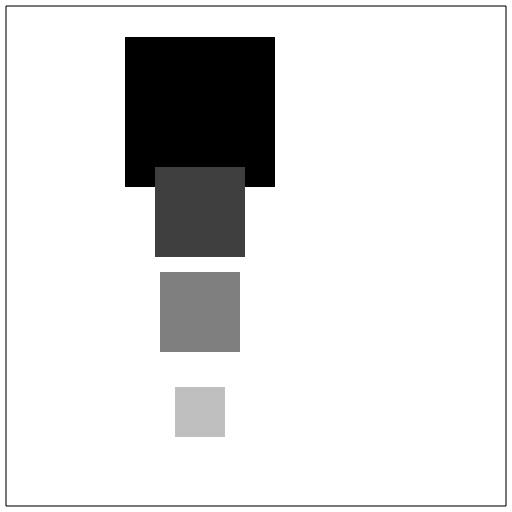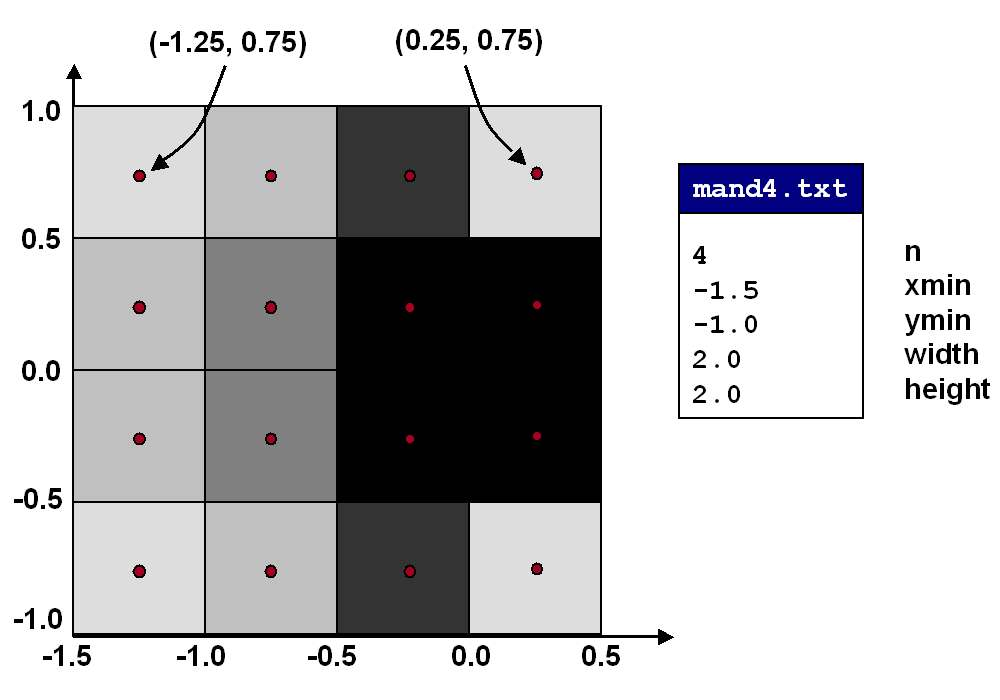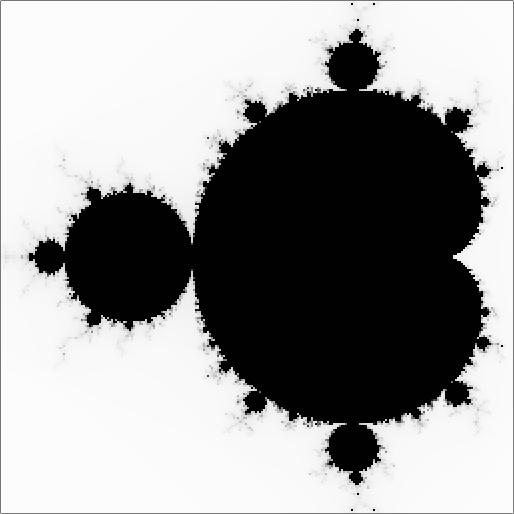COS 126 Mandelbrot Set Programming Assignment Due: Wednesday

Write a C program to generate a Flying Turtle Graphics program that will plot the Mandelbrot set.

The Mandelbrot set is a specific set of points on the plane with many fascinating properties. One can determine whether or not a point (x, y) is in the Mandelbrot set by performing the following calculation: start with r = x and s = y, then enter into a loop which resets r to r*r - s*s + x and s to 2*r*s + y (using the old values of r and s in both of these update formulae). If this sequence remains bounded (neither r nor s goes to infinity) then the point (x, y) is in the Mandelbrot set; otherwise, if the sequence diverges to infinity, it is not. If you plot the points in the Mandelbrot set black, and those not in the set white, a strange and wondrous pattern emerges, roughly within the 2.0-by-2.0 box centered at (-0.5, 0). For a more dramatic picture, you will replace the white points with color gradations, depending on the number of iterations needed to discover that the point is not in the set. These colors reveal the striking detail of the terrain just outside the Mandelbrot set.

Part 1: Mandelbrot computation. Your first task is to write a function that determines whether or not the point (x, y) is in the set. The mathematical definition of the Mandelbrot set is precise, but is computationally unappealing, since we would have to perform an infinite number of iterations to determine whether a single point is in the set! We are rescued by the following mathematical fact: if r*r + s*s ever gets to be greater than 4, then the sequence will surely diverge off to infinity, and the point (x, y) is not in the set. There is no simple test that would enable us to conclude that (x, y) is surely in the set, but if the number of iterations exceeds 255, the point is extremely likely to be in the set.

Write a function int mandel(double x, double y) that performs the Mandelbrot computation on the point (x, y). It returns the number of iterations needed to discover that (x, y) is not in the set, or 255 if 255 or more iterations are required (this will be the case for all points in the set, and perhaps a few others). Using this function, you will produce gray-scale and color images of the Mandelbrot set.

Part 2: gray-scale image. To produce the gray-scale image of the Mandelbrot set, you would need to perform the Mandelbrot calculation at every point in the plane. You cannot plot an infinite number of points, so you will restrict attention to a particular rectangular region (read in as input), and plot a "sample" `n`-by-`n` grid of evenly spaced points from the continuum. Large values of n will produce higher resolution images, at the cost of more computation. Your program will read in the integer n from standard input. It will also read in the rectangular region of interest given by the x and y coordinates of the lower left endpoint (xminand ymin) and the dimension (width and height) endpoints of the rectangular region of interest. The interesting part of the Mandelbrot set occurs in a 2.0-by-2.0 box centered at (-0.5, 0.0): the data in mand32.txt has n = 32, (xmin, ymin) = (-1.5, -1.0), and dimension (width, height) = (2.0, 2.0). The other data files zoom in on different regions.

Flying turtle graphcs primer. The flying turtle graphics language from Lecture P2 has several commands for controlling the actions of an artistic turtle in a 512-by-512 box. We review the three commands needed to complete this assignment.

• C r g b:   Change the current pen color in RGB units. The three color parameters must be between 0.0 and 1.0. Black, white, red, green, and blue are (0, 0, 0), (1, 1, 1), (1,0,0), (0,1,0), and (0,0,1), respectively. (0.5, 0.5, 0.5) is a shade of gray; (1, 0, 1) is magenta.

• F x y:   Fly to location (x, y).

• S size:   Drop a spot of the specified size, centered at the current (x, y) location, in the current pen color.
• For example, the following turtle graphics program plots four squares of different sizes in various shades of gray.
 ```C 0.00 0.00 0.00 F 200.0 400.0 S 150.0 C 0.25 0.25 0.25 F 200.0 300.0 S 90.0 C 0.50 0.50 0.50 F 200.0 200.0 S 80.0 C 0.75 0.75 0.75 F 200.0 100.0 S 50.0 ```To understand how it works, put this program into a text file called test.tur and enter the command

```turtle < test.tur > test.ps
```
to create the PostScript file test.ps. You can now view or print this file from your operating system.

Back to Part 2. Your task is to write a C program that produces the turtle graphics program as output, except with different arguments for the C, F, and S commands (and many more calls on them). One of your challenges is to translate and scale the plane coordinates from the rectangle `(xmin, ymin, width, height)` to the 512x512 box with endpoints (0, 0) and (512, 512). Also, be sure to adjust the size parameter so that the squares just touch without overlapping. Choose the gray-scale for a point according to the formula 1.0 - t/255.0, where t is the number of iterations in the Mandelbrot calculation for that point, and use this value for all three arguments to the C command. This ensures that all points in the Mandelbrot set are colored black. Points just outside the set will be colored in shades of gray. You should perform the Mandelbrot at the center of each grid-box, and use this value to determine the gray-scale level, i.e., at the circled points in the figure below.When you run your C program, read the input from one of the data files, pipe its output through the turtle graphics engine, and redirect its output to a PostScript file with the command

```Windows:  mandgray < mand4.txt | turtle > mandgray4.ps
Unix:     a.out    < mand4.txt | turtle > mandgray4.ps
```
The file mandgray4.ps now contains a PostScript program! To debug, first make certain that your C program produces a valid turtle graphics program exactly like the sample program, except with different calls to F, C, and S.

Part 3: color image. The third part of the assignment is to generate a color version of the Mandelbrot set. For each point, the Mandelbrot calculation returns a value between 0 and 255. Depending on this value, you will plot the point in a different color using the C command. The following turtle graphics snippet plots a square of size 64, centered at (128, 128) in a shade of magenta.

```C 0.800 0.000 0.800
F 128.0 128.0
S 64.0
```

The input files supply a table that gives the three red-green-blue parameters for each possible iteration count between 0 and 255. If the Mandelbrot computation takes t iterations, then use line t from the table (start counting from 0) as the 3 arguments for the C command. To do this, define three 256-element arrays (or one 2-dimensional array) and initialize it by reading the table from standard input using scanf(). These values will occur after n, xmin, ymin, width, and height in the input file.

Submission. Submit the following files: readme.txt, mandgray.c, and mandcolor.c.

Extra credit. There are limitless opportunities to avoid boredom here and earn extra credit. For example, create your own data files that zoom way in on other interesting parts of the set, near "edges" and sharp corners, and experiment with different color maps. Or experiment with different update formulae for r and s.This assignment was adapted by R. Sedgewick and M. Weiksner from the book A Turing Omnibus by A. Dewdney. Modified slightly by Adam Finkelstein and Kevin Wayne.